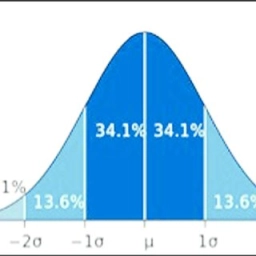# 3 sigma

Students' performance scores in a statistic test have a mean of 70 and a standard deviation of 4.0. The scores obtained can be modeled by a normal distribution. Find the probability that the score of a randomly selected student is

i. more than 80 marks
ii. less than 65 marks

Find also the number of students who scored above 80 marks if a total of 1200 students sat for the test.

a =  0.0062
b =  0.1056
c =  7

### Step-by-step explanation:Did you find an error or inaccuracy? Feel free to write us. Thank you!

Tips for related online calculators
Looking for a statistical calculator?
Do you want to round the number?
Would you like to compute the count of combinations?